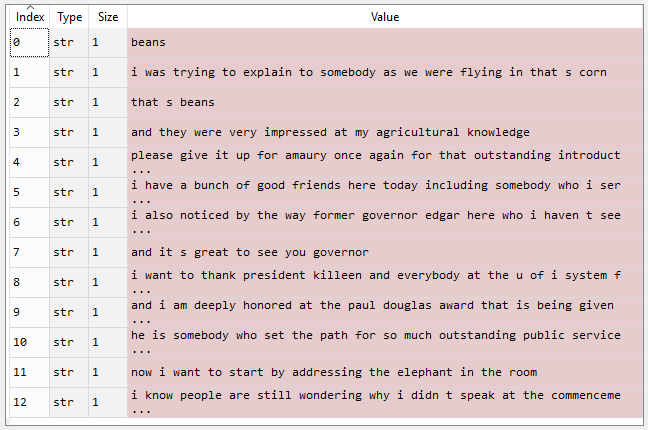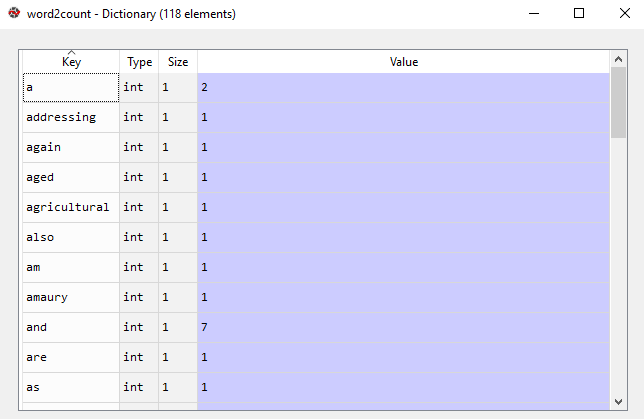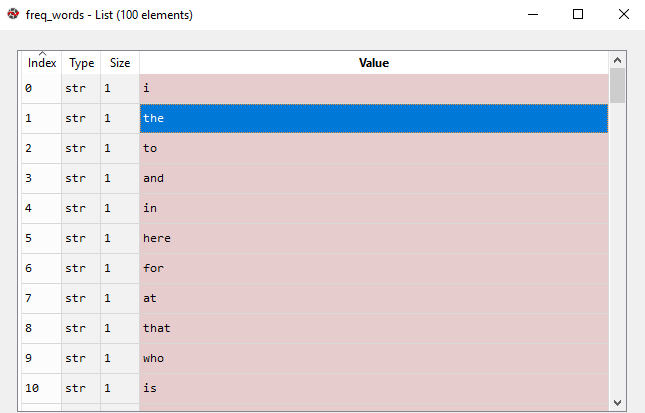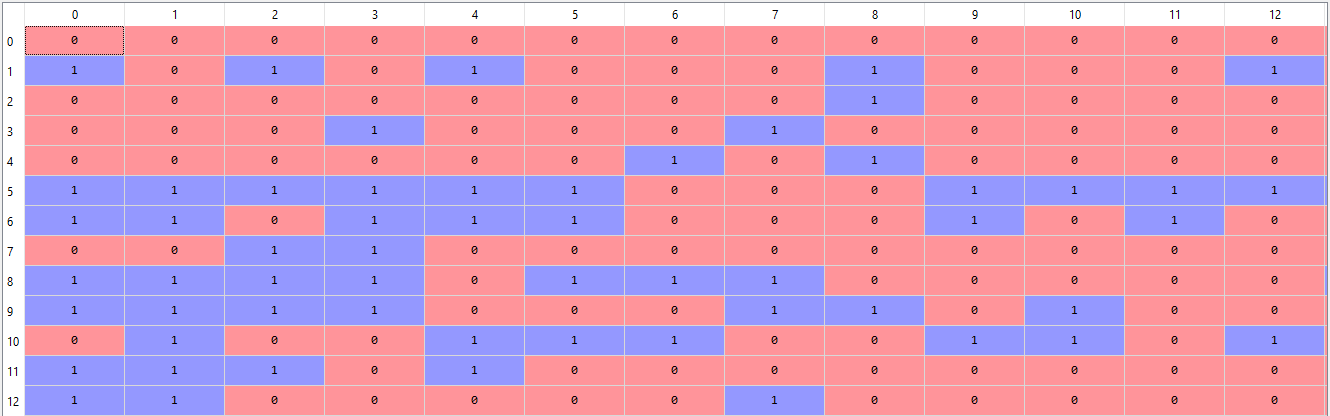# Bag of words (BoW) model in NLP

• Difficulty Level : Medium
• Last Updated : 08 Mar, 2019

In this article, we are going to discuss a Natural Language Processing technique of text modeling known as Bag of Words model. Whenever we apply any algorithm in NLP, it works on numbers. We cannot directly feed our text into that algorithm. Hence, Bag of Words model is used to preprocess the text by converting it into a bag of words, which keeps a count of the total occurrences of most frequently used words.

This model can be visualized using a table, which contains the count of words corresponding to the word itself.

Attention reader! Don’t stop learning now. Get hold of all the important Machine Learning Concepts with the Machine Learning Foundation Course at a student-friendly price and become industry ready.

Applying the Bag of Words model:

Let us take this sample paragraph for our task :

Beans. I was trying to explain to somebody as we were flying in, that’s corn. That’s beans. And they were very impressed at my agricultural knowledge. Please give it up for Amaury once again for that outstanding introduction. I have a bunch of good friends here today, including somebody who I served with, who is one of the finest senators in the country, and we’re lucky to have him, your Senator, Dick Durbin is here. I also noticed, by the way, former Governor Edgar here, who I haven’t seen in a long time, and somehow he has not aged and I have. And it’s great to see you, Governor. I want to thank President Killeen and everybody at the U of I System for making it possible for me to be here today. And I am deeply honored at the Paul Douglas Award that is being given to me. He is somebody who set the path for so much outstanding public service here in Illinois. Now, I want to start by addressing the elephant in the room. I know people are still wondering why I didn’t speak at the commencement.

Step #1 : We will first preprocess the data, in order to:

• Convert text to lower case.
• Remove all non-word characters.
• Remove all punctuations.
 `# Python3 code for preprocessing text``import` `nltk``import` `re``import` `numpy as np`` ` `# execute the text here as :``# text = """ # place text here  """``dataset ``=` `nltk.sent_tokenize(text)``for` `i ``in` `range``(``len``(dataset)):``    ``dataset[i] ``=` `dataset[i].lower()``    ``dataset[i] ``=` `re.sub(r``'\W'``, ``' '``, dataset[i])``    ``dataset[i] ``=` `re.sub(r``'\s+'``, ``' '``, dataset[i])`

Output:Preprocessed text

You can further preprocess the text to suit you needs.

Step #2 : Obtaining most frequent words in our text.

We will apply the following steps to generate our model.

• We declare a dictionary to hold our bag of words.
• Next we tokenize each sentence to words.
• Now for each word in sentence, we check if the word exists in our dictionary.
• If it does, then we increment its count by 1. If it doesn’t, we add it to our dictionary and set its count as 1.
 `# Creating the Bag of Words model``word2count ``=` `{}``for` `data ``in` `dataset:``    ``words ``=` `nltk.word_tokenize(data)``    ``for` `word ``in` `words:``        ``if` `word ``not` `in` `word2count.keys():``            ``word2count[word] ``=` `1``        ``else``:``            ``word2count[word] ``+``=` `1`

Output:Bag of Words dictionary

In our model, we have a total of 118 words. However when processing large texts, the number of words could reach millions. We do not need to use all those words. Hence, we select a particular number of most frequently used words. To implement this we use:

 `import` `heapq``freq_words ``=` `heapq.nlargest(``100``, word2count, key``=``word2count.get)`

where 100 denotes the number of words we want. If our text is large, we feed in a larger number.100 most frequent words

Step #3 : Building the Bag of Words model
In this step we construct a vector, which would tell us whether a word in each sentence is a frequent word or not. If a word in a sentence is a frequent word, we set it as 1, else we set it as 0.
This can be implemented with the help of following code:

 `X ``=` `[]``for` `data ``in` `dataset:``    ``vector ``=` `[]``    ``for` `word ``in` `freq_words:``        ``if` `word ``in` `nltk.word_tokenize(data):``            ``vector.append(``1``)``        ``else``:``            ``vector.append(``0``)``    ``X.append(vector)``X ``=` `np.asarray(X)`

Output:BoW model

My Personal Notes arrow_drop_up